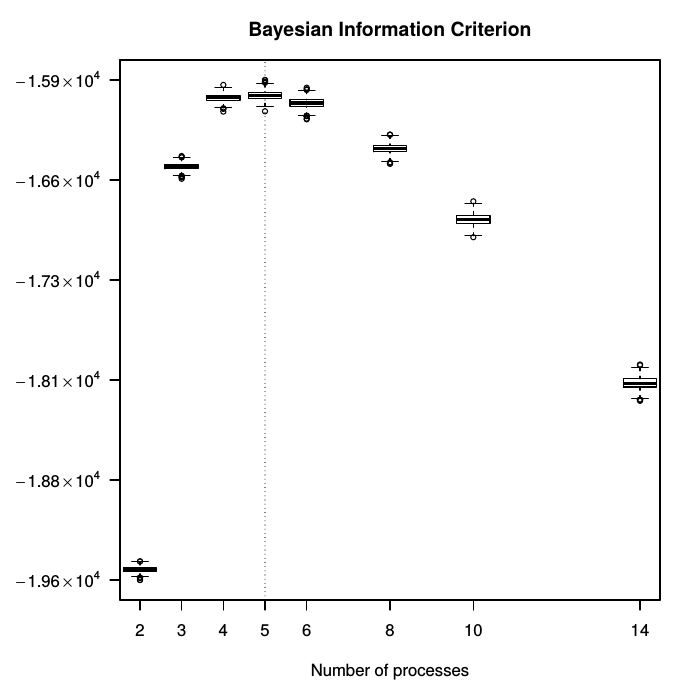# signeR

## 1: Introduction

Motivation: Cancer is an evolutionary process driven by continuous acquisition of genetic variations in individual cells. The diversity and complexity of somatic mutational processes is a conspicuous feature orchestrated by DNA damage agents and repair processes, including exogenous or endogenous mutagen exposures, defects in DNA mismatch repair and enzymatic modification of DNA. The identification of the underlying mutational processes is central to understanding of cancer origin and evolution.

The signeR package focuses on the estimation and further analysis of mutational signatures. The functionalities of this package can be divided into three categories. First, it provides tools to process VCF files and generate matrices of SNV mutation counts and mutational opportunities, both defined according to a 3bp context (mutation site and its neighbouring 3' and 5' bases). Second, these count matrices are considered as input for the estimation of the underlying mutational signatures and the number of active mutational processes. Third, the package provides tools to correlate the activities of those signatures with other relevant information such as clinical data, in order to draw conclusions about the analysed genome samples, which can be useful for clinical applications. These include the Differential Exposure Score and the a posteriori sample classification.

Although signeR is intended for the estimation of mutational signatures, it actually provides a full Bayesian treatment to the non-negative matrix factorisation (NMF) model. Further details about the method can be found in Rosales & Drummond et al., 2016 (see section 6.1 below).

This vignette briefly explains the use of signeR through examples.

## 2: Installation

To install signeR, start R and enter:

```source("https://bioconductor.org/biocLite.R")
biocLite("signeR")
```

Once installed the library can be loaded as

```library(signeR)
```

OS X users might experience compilation errors due to missing gfortran libraries. Please read this section.

## 3: Preparing the input

signeR takes as input a count matrix of samples x features. Each feature is usually a SNV mutation within a 3bp context (96 features, 6 types of SNV mutations and 4 possibilities for the bases at each side of the SNV change). Optionally, an opportunity matrix can also be provided containing the count frequency of the features in the whole analyzed region for each sample. Although not required, this argument is highly recommended because it allows signeR to normalize the features frequency over the analyzed region.

Input matrices can be read both from a VCF or a tab-delimited files, as described next.

### 3.1: Input from VCF

The VCF file format is the most common format for storing genetic variations, the signeR package includes a utility function for generating a count matrix from the VCF:

```library(VariantAnnotation)

# BSgenome, equivalent to the one used on the variant call
library(BSgenome.Hsapiens.UCSC.hg19)

mut <- genCountMatrixFromVcf(BSgenome.Hsapiens.UCSC.hg19, vcfobj)
```

This function will generate a matrix of mutation counts for each sample in the provided VCF. The opportunity matrix can also be generated from the reference genome (hg19 in the following case):

```library(rtracklayer)

target_regions <- import(con="/path/to/a/target.bed", format="bed")
opp <- genOpportunityFromGenome(BSgenome.Hsapiens.UCSC.hg19,
target_regions, nsamples=nrow(mut))
```

Where `target.bed` is a bed file containing the genomic regions analyzed by the variant caller.

### 3.2: Input from tab-delimited file

By convention, the input file should be tab-delimited with sample names as row names and features as column names. Features should be refered in the format "base change:triplet", e.g. "C>A:TCG", as can be seen in the example below. Similarly, the opportunity matrix can be provided in a tab-delimited file with the same structure as the mutation counts file. An example of the required matrix format can be seen here.

This tutorial uses as input the 21 breast cancer dataset described in Nik-Zainal et al 2012. For the sake of convenience this dataset is included with the package and can be accessed by using the `system.file` function:

```mut <- read.table(system.file("extdata","21_breast_cancers.mutations.txt",
package="signeR"))
```

## 4: Estimating the number of mutational processes and their signatures

signeR takes a count matrix as its only required parameter, but the user can provide an opportunity matrix as well. The algorithm allows the assessment of the number of signatures by three options, as follows.

1. signeR detects the number of signatures at run time by considering the best NMF factorisation rank between 1 and min(G, K)-1, with G = number of genomes and K = number of features (i.e. 96):
```signatures <- signeR(M=mut, Opport=opp)
```
2. The user can give a interval of the possible numbers of signatures as the parameter nlim. signeR will calculate the optimal number of signatures within this range, for example:
```signatures <- signeR(M=mut, Opport=opp, nlim=c(2,11))
```
3. Finally, signeR can also be run by passing the number of signatures as the parameter nsig. In this setting, the algorithm is faster. For example, the following command will make signeR consider only the rank N=5 to estimate the signatures and their exposures:
```signatures <- signeR(M=mut, Opport=opp, nsig=5, main_eval=100, EM_eval=50, EMit_lim=20)
```

The parameters `testing_burn` and `testing_eval` control the number of iterations used to estimate the number of signatures (default value is 1000 for both parameters). There are other arguments that may be passed on to signeR. Please have a look at signeR's manual, issued by typing `help(signeR)`.

Whenever signeR is left to decide which number of signatures is optimal, it will search for the rank Nsig that maximizes the median Bayesian Information Criterion (BIC). After the processing is done, this information can be plotted by the following command:

```BICboxplot(signatures)
```Boxplot of BIC values, showing that the optimal number of signatures for this dataset is 5.

## 5: Results and further analyses

### 5.1: Plot the MCMC paths for the NMF parameters (P and E matrices)

The following instruction plots the MCMC sampled paths for each entry of the signature matrix P and their exposures, i.e. the E matrix. Only post-burnin paths are available for plotting. Those plots are useful for checking if entries have leveled off, reflecting the sampler convergence.

```Paths(signatures\$SignExposures)
```